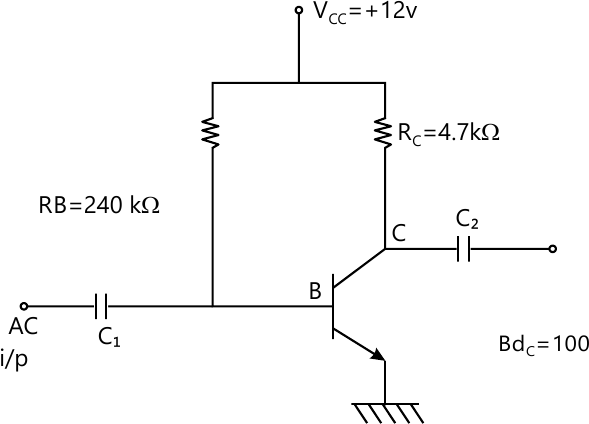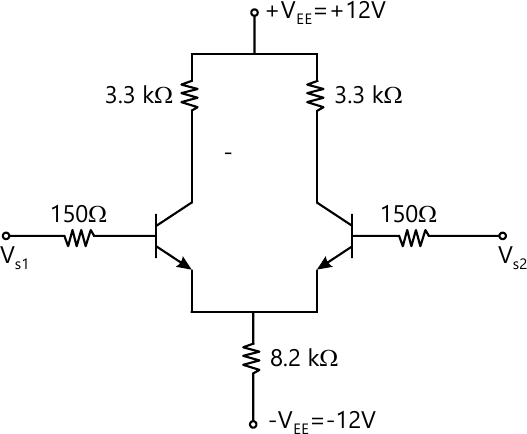MORE IN Analog & Digital Circuits
MU Information Technology (Semester 3)
Analog & Digital Circuits
December 2013
Total marks: --
Total time: --
INSTRUCTIONS
(1) Assume appropriate data and state your reasons
(2) Marks are given to the right of every question
(3) Draw neat diagrams wherever necessary

Solve any four:-
1 (a) Find out relations between ?, ? and ? as current amplification factors for CE, CB, CC configurations.
5 M
1 (b) State ideal and practical characteristics of Op-Amp.
5 M
1 (c) Convert following decimal number to Binary, Octal, Herl-equivalent and gray code.
(i) (306.8)10 (ii) (147.8)10
5 M
1 (d) Add (57)10 and (26)10 in BCD
5 M
1 (e) Convert S-R flip-flop to D-flip-flop
5 M
1 (f) Explain parallel input, serial output shift register.
5 M

2 (a) Determine the following for the fixed bias.
(i) IBO and ICQ
(ii) VCEQ
(iii) VB and VC
(iv) VBC8 M
2 (b) For the differential amplifier as shown below calculate: -
(i) Operating points
(ii) Voltage gain
(iii) Input Impedance
(iv) Output impedance8 M
2 (c) Design a circuit to V0=15 (V1-V2) where V1 and V2 are input voltage to Op-Amp.
4 M

3 (a) What are different methods used to improve CMRR in differential amplifier?
8 M
3 (b) Design a differentiator to differentiate an input signal that varies in frequency from 10 Hz to about 5 KHz.
8 M
3 (c) Explain Instrumentation amplifier using 3 Op-Amps.
4 M

4 (a) Design an astable multivibrator for an output frequency of 1KHz and duty cycle 40%.
6 M
4 (b) Minimize the following expression using K-map and realize using the gates.
Y=?m(1,2,9,10,11,15,15)
6 M
4 (c) Design 2-bit magnitude comparator using basic gates.
8 M

5 (a) Implement the following function using 8: 1 MUX
f(A, B, C, D)=?m(2,4,5,7,10,15)
6 M
5 (b) Implement full adder using demultiplexer.
6 M
5 (c) Design the divide by 7 (mod-7) asynchronous up-counter using J-K flip flop. Also state difference between synchronous and asynchronous counter.
8 M

6 (a) Explain universal shift register and its applications.
6 M
6 (b) Explain VHDL
4 M
6 (c) What is Zener shunt regulator? Explain and derive stability factor.
4 M
6 (d) Realize following using only NAND gates.
Y=(AB+BC)C.
6 M

More question papers from Analog & Digital Circuits